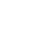# CombiMirror Final

If you prefer to just copy/paste flames into JWildfire  see this post here  which allows that.

So, what is it all about: This variation can mirror a flame at all 3 axis (x,y,z) and has a point mirror option. Each mirror has also a move slider, that does a move along an axis (like post_mirror_wf). And finally there are color sliders for each mirror types, that can easily color a flame. Below is the code to enter into custom_wf or custom_wf_full. Or you can download the java file and add it to your custom variations, for the custom variation loader, see the information about custom variations here.

The mirror parameter has sliders that can fade in or fade out one mirror effect. 0 means mirror is faded out, 1 means mirror is on, 2 means mirror is faded out in the other direction. The color sliders works in a range between 0 and 1. The move sliders can have any value, positive, 0 or negative

Parameter list:

vmirror Vertical mirror (y-axis)

vmove moving along y-axis

hmirror Horizontal mirror (x-axis)

hmove moving along x-axis

zmirror Depth mirror (z-axis)

zmove moving along z-axis

pmirror Mirror around center point

pmovex moving along x direction

pmovey moving along y direction

vcolorshift Vertical axis

hcolorshift Horizontal axis

zcolorshift Z-axis

pcolorshift Point

```/*
JWildfire - an image and animation processor written in Java
This is free software; you can redistribute it and/or modify it under the terms of the GNU Lesser
This software is distributed in the hope that it will be useful, but WITHOUT ANY WARRANTY; without
even the implied warranty of MERCHANTABILITY or FITNESS FOR A PARTICULAR PURPOSE. See the GNU
Lesser General Public License for more details.
You should have received a copy of the GNU Lesser General Public License along with this software;
if not, write to the Free Software Foundation, Inc., 51 Franklin St, Fifth Floor, Boston, MA
02110-1301 USA, or see the FSF site: http://www.fsf.org.
*/
package org.jwildfire.create.tina.variation;
import org.jwildfire.create.tina.base.XForm;
import org.jwildfire.create.tina.base.XYZPoint;
import org.jwildfire.base.mathlib.Complex;
import static org.jwildfire.base.mathlib.MathLib.fmod;
/*
Combination of vertical, horizontal, z mirror and point mirror
All parameter works in range of 0..1
by Thomas Michels and the great support by Brad Stefanov
*/
public class Combimirror_Func extends VariationFunc {
private static final long serialVersionUID = 1L;
private static final String PARAM_VMIRROR = vmirror;
private static final String PARAM_VMOVE = vmove;
private static final String PARAM_HMIRROR = hmirror;
private static final String PARAM_HMOVE = hmove;
private static final String PARAM_ZMIRROR = zmirror;
private static final String PARAM_ZMOVE = zmove;
private static final String PARAM_POINT = pmirror;
private static final String PARAM_POINTX = pmovex;
private static final String PARAM_POINTY = pmovey;
private static final String PARAM_VCOLORSHIFT = vcolorshift;
private static final String PARAM_HCOLORSHIFT = hcolorshift;
private static final String PARAM_ZCOLORSHIFT = zcolorshift;
private static final String PARAM_PCOLORSHIFT = pcolorshift;
private static final String[] paramNames = { PARAM_VMIRROR, PARAM_VMOVE, PARAM_HMIRROR,
PARAM_HMOVE, PARAM_ZMIRROR, PARAM_ZMOVE, PARAM_POINT, PARAM_POINTX, PARAM_POINTY, PARAM_VCOLORSHIFT, PARAM_HCOLORSHIFT, PARAM_ZCOLORSHIFT, PARAM_PCOLORSHIFT };
private double mv1 = 1.0;
private double mv2 = 0.0;
private double mh1 = 0.0;
private double mh2 = 0.0;
private double mz1 = 0.0;
private double mz2 = 0.0;
private double mp = 0.0;
private double mpx = 0.0;
private double mpy = 0.0;
private double vcolorshift = 0.0;
private double hcolorshift = 0.0;
private double zcolorshift = 0.0;
private double pcolorshift = 0.0;
@Override
public void transform(FlameTransformationContext pContext, XForm pXForm, XYZPoint pAffineTP, XYZPoint pVarTP, double pAmount) {
Complex z = new Complex(pAffineTP.x, pAffineTP.y);
Complex z2 = new Complex(pAffineTP.z, pAffineTP.y);
z.Scale(pAmount);
pVarTP.x = z.re;
pVarTP.y = z.im;
pVarTP.z = z2.re;
//Mirror around center point
if (pContext.random() > mp/2){
pVarTP.x = -pVarTP.x + mpx;
pVarTP.y = -pVarTP.y + mpy;
pVarTP.color = fmod(pVarTP.color + pcolorshift, 1.0);
}
//Mirror along vertical axis
if (pContext.random() < mv1/2){
pVarTP.x = -pVarTP.x + mv2;
pVarTP.color = fmod(pVarTP.color + vcolorshift, 1.0);
}
//Mirror along horizontal axis
if (pContext.random() < mh1/2){
pVarTP.y = -pVarTP.y + mh2;
pVarTP.color = fmod(pVarTP.color + hcolorshift, 1.0);
}
//Mirror along Z axis
if (pContext.random() < mz1){
pVarTP.z = -pVarTP.z + mz2;
pVarTP.color = fmod(pVarTP.color + zcolorshift, 1.0);
}
//if (pContext.isPreserveZCoordinate()) {
//pVarTP.z += pAmount * pAffineTP.z;
//}
}
@Override
public String[] getParameterNames() {
return paramNames;
}
@Override
public Object[] getParameterValues() {
return new Object[] {mv1, mv2, mh1, mh2, mz1, mz2, mp, mpx, mpy, vcolorshift, hcolorshift, zcolorshift, pcolorshift};
}
@Override
public void setParameter(String pName, double pValue) {
if (PARAM_VMIRROR.equalsIgnoreCase(pName))
mv1 = pValue;
else if (PARAM_VMOVE.equalsIgnoreCase(pName))
mv2 = pValue;
else if (PARAM_HMIRROR.equalsIgnoreCase(pName))
mh1 = pValue;
else if (PARAM_HMOVE.equalsIgnoreCase(pName))
mh2 = pValue;
else if (PARAM_ZMIRROR.equalsIgnoreCase(pName))
mz1 = pValue;
else if (PARAM_ZMOVE.equalsIgnoreCase(pName))
mz2 = pValue;
else if (PARAM_POINT.equalsIgnoreCase(pName))
mp = pValue;
else if (PARAM_POINTX.equalsIgnoreCase(pName))
mpx = pValue;
else if (PARAM_POINTY.equalsIgnoreCase(pName))
mpy = pValue;
else if (PARAM_VCOLORSHIFT.equalsIgnoreCase(pName))
vcolorshift = pValue;
else if (PARAM_HCOLORSHIFT.equalsIgnoreCase(pName))
hcolorshift = pValue;
else if (PARAM_ZCOLORSHIFT.equalsIgnoreCase(pName))
zcolorshift = pValue;
else if (PARAM_PCOLORSHIFT.equalsIgnoreCase(pName))
pcolorshift = pValue;
else
throw new IllegalArgumentException(pName);
}
@Override
public String getName() {
return combimirror;
}
}```

•zip
• Version
• File Size 1.66 KB
• File Count 1
• Create Date February 2, 2020
• Categories
• Tags
• ### Attached Files

• FileAction
combimirrorfinal.zip